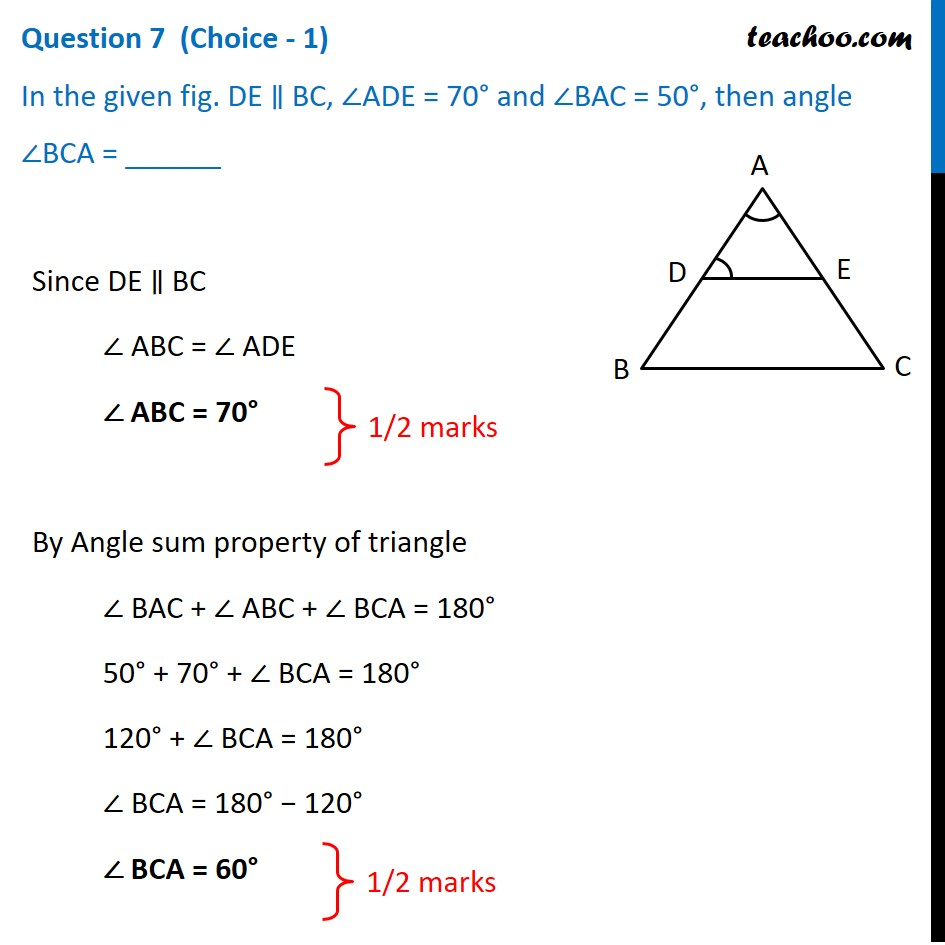## In the given fig. DE ∥ BC, ∠ADE = 70° and ∠BAC = 50°, then angle ∠BCA = ______1. Class 10
2. Solutions of Sample Papers for Class 10 Boards
3. CBSE Class 10 Sample Paper for 2021 Boards - Maths Basic

Transcript

Question 7 (Choice - 1) In the given fig. DE ∥ BC, ∠ADE = 70° and ∠BAC = 50°, then angle ∠BCA = ______Since DE ∥ BC ∠ ABC = ∠ ADE ∠ ABC = 70° By Angle sum property of triangle ∠ BAC + ∠ ABC + ∠ BCA = 180° 50° + 70° + ∠ BCA = 180° 120° + ∠ BCA = 180° ∠ BCA = 180° − 120° ∠ BCA = 60° 1/2 marks 1/2 marks

CBSE Class 10 Sample Paper for 2021 Boards - Maths Basic

Class 10
Solutions of Sample Papers for Class 10 Boards